## why the way solution is written affects odetest re...

I found a new strange thing with odetest that I have not seen before.

Would you say that these two solutions are the same or not?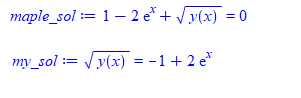They look the same to me.  One just have all the terms on one side, that is all.

Why would Maple odetest verify the first one but not the second? I did not know that all terms have to be on one side before. Is this documented somewhere?

 > restart;
 > interface(version);> Physics:-Version();> ode:=diff(y(x), x) - 2*y(x) = 2*sqrt(y(x)); ic:=[y(0)=1]; maple_sol:=dsolve([ode,op(ic)],implicit); odetest(maple_sol,[ode,op(ic)]) assuming x>0> #now move some terms to one side, and odetest no longer verifies it my_sol:=y(x)^(1/2) = -1+2*exp(x); odetest(my_sol,[ode,op(ic)]) assuming x>0> #the solution is to put all terms on one side my_sol:=y(x)^(1/2) = -1+2*exp(x); odetest(rhs(my_sol)-lhs(my_sol)=0,[ode,op(ic)]) assuming x>0>

I've been studying the  drawing  of graph lately .    One of the themes is  1-planar graph .

A 1-planar graph is a graph that can be drawn in the Euclidean plane in such a way that each edge has at most one crossing point,  where it crosses a single additional edge. If a 1-planar graph, one of the most natural generalizations of planar graphs, is drawn that way, the drawing is called a 1-plane graph or 1-planar embedding of the graph.I know it is NP hard to determine whether a graph is a 1-planar . My idea is to take advantage of some mathematical software to provide some roughly and  intuitive understanding before determining .

Now,  the layout of vertices or edges becomes important.  The drawing of a plane graph is a good example.

DrawGraph(G1)
DrawGraph(G1,style=planar)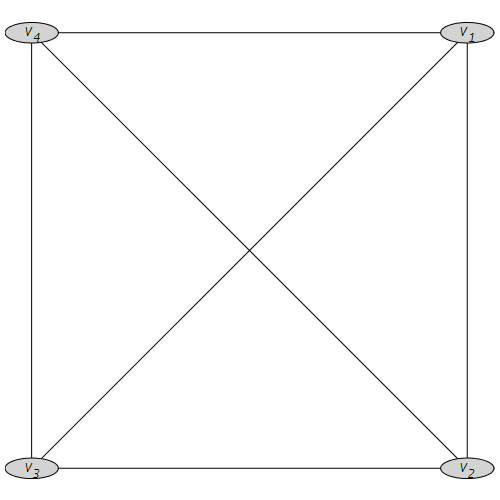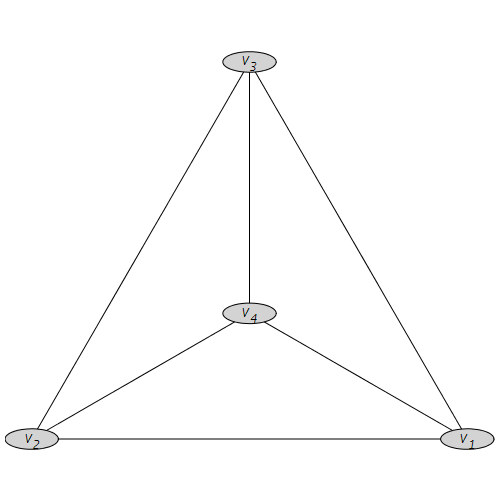K5 := CompleteGraph(5);
DrawGraph(K5);
vp:=[[-1,0],[1,0],[-0.2,0.5],[0.2,0.5],[0,1]];
SetVertexPositions(K5,vp);  #modified the vertex position

DrawGraph(K5);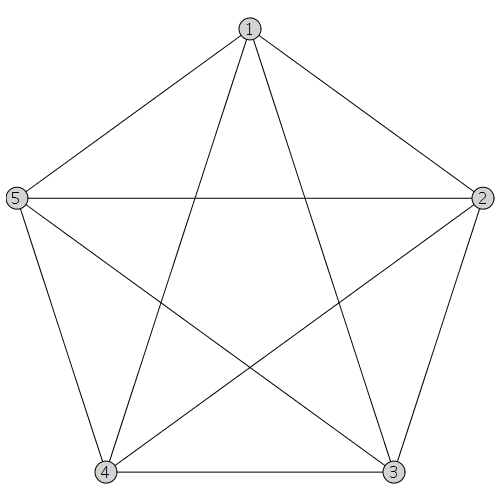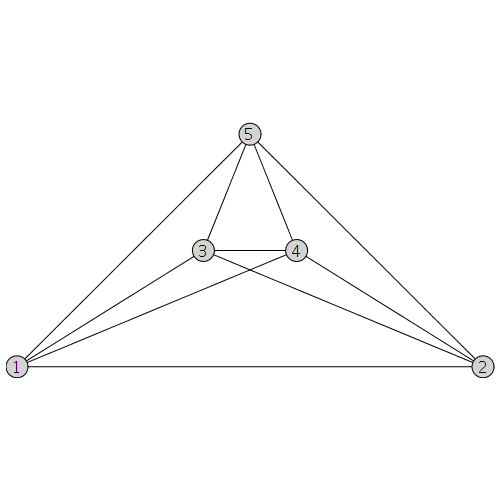My problem is that I see that  Maple2020 has updated a lot of layouts about DrawGraph  graph theory backpack , and I don’t know which ones are working towards the least possible number of crossing of  each edges of graph .

Some links that may be useful:

https://de.maplesoft.com/products/maple/new_features/Maple2020/graphtheory.aspx

https://de.maplesoft.com/support/help/Maple/view.aspx?path=GraphTheory/SetVertexPositions

I think the software can improve some calculations related to topological graph theory, such as crossing number of graph, etc.

## Maple UI is tiny on a high dpi screen in Linux...

I'm using Maple 2020 in Linux (Ubuntu 20.04). The screen resolution is 2560×1440, so I have system-wide setting of 200% scaling for all apps, but unfortunately Maple doesn't adopt the system setting and UI elements are tiny. To make it tolerable, in "Tools - Options - Interface", I've set Default Zoom to be 200% and checked the box "Large toolbar icons". However, menus (File, Edit, View etc.) and dialog boxes are still tiny.

Am I right that the Maple GUI is written in Java / Swing? I've heard that Java 9 has better support for high dpi scaling. Does the default Maple installation use Java 9?

## Can I solve one equation in one variable in parall...

Dear All,
I want to solve a highly nonlinear equation in one variable. The equation includes some undetermined parameters. The running time is long in serial programming. Can I help me to execute the following command in parallel? I know that the ‘solve’ command is not thread-safe.

solve(Eq1, beta);

Let me know solve the problem, if the number of equations is larger than one, as below:
solve({eq1,eq2,eq3},{beta1,beta2,beta3});
Best wishes

## Bug in modp1(('Embed')(...))...

Hi all,

There is issue with modp1(('Embed')(...)) function.

bug_embed.mw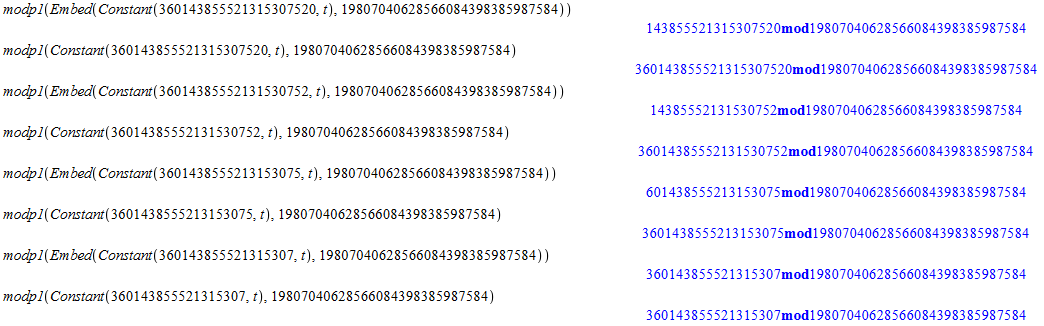As you can see in lines 1, 3, 5 Embed function in combining with Constant cutting several digits from argument.

Thank you.

## How can I solve two equations?...

How I can find the coefficient an, and bn according to the following solution?

the coefficients an and bn can be found by solving the
two linear equations that come from V = V at eta=eta and
V = V at eta=eta, and comparing with following Eq in each
case.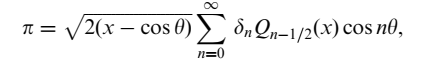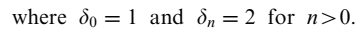Best

2.mw

## Bug in modp1(('Rem')(...))...

Hi there.

It seems like a bug in modp1(('Rem')(...)) with large polynomials with large coefficients.

bug_rem.mw

It needs the file polys.m there:

https://dropmefiles.com/9fATR

File polys.m too big for this forum so I used dropmefiles.

Polys.m contain two polynomials x and f_t with large degrees: degree(f_t) = m = 50021, degree(x) = 2*m - 2 = 100040 and large coefficients up to 2^N, where N = ceil(m / 2)+2 = 25013.

I just compute rem(x,f_t) mod 2^N.

As you can see in the first part of doc bug_rem.mw I decreased coefficients of polynomials by additional mod 2^N (with Embed function), where N = floor(N / 2) = 12506. WIth these decreased polynomials and decreased N modp1(('Rem')(...)) function works well and use maximum about 2.5 Gb of RAM.

But in the second part of doc bug_rem.mw with original polynomials and N = 25013 modp1(('Rem')(...)) use maximum about 3.5 Gb of RAM and crash with error:

Error, Maple was unable to allocate enough memory to complete this computation.  Please see ?alloc

This is strange and looks like a bug considering that the test server has 48 Gb of RAM.

Is it a bug or modp1(('Rem')(...)) just need more than 48 Gb of RAM?

How many RAM it needs for this computation?

Thank you